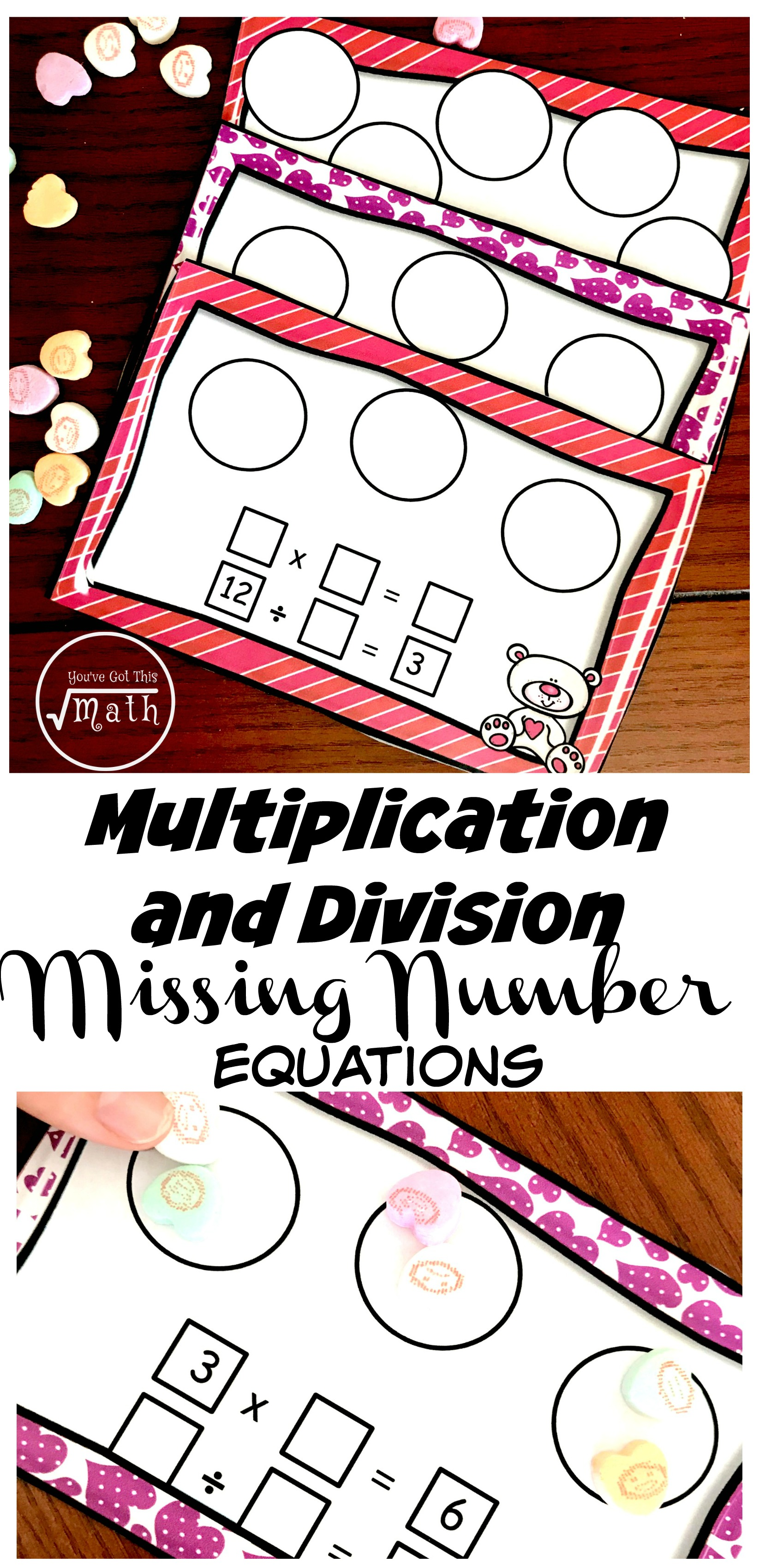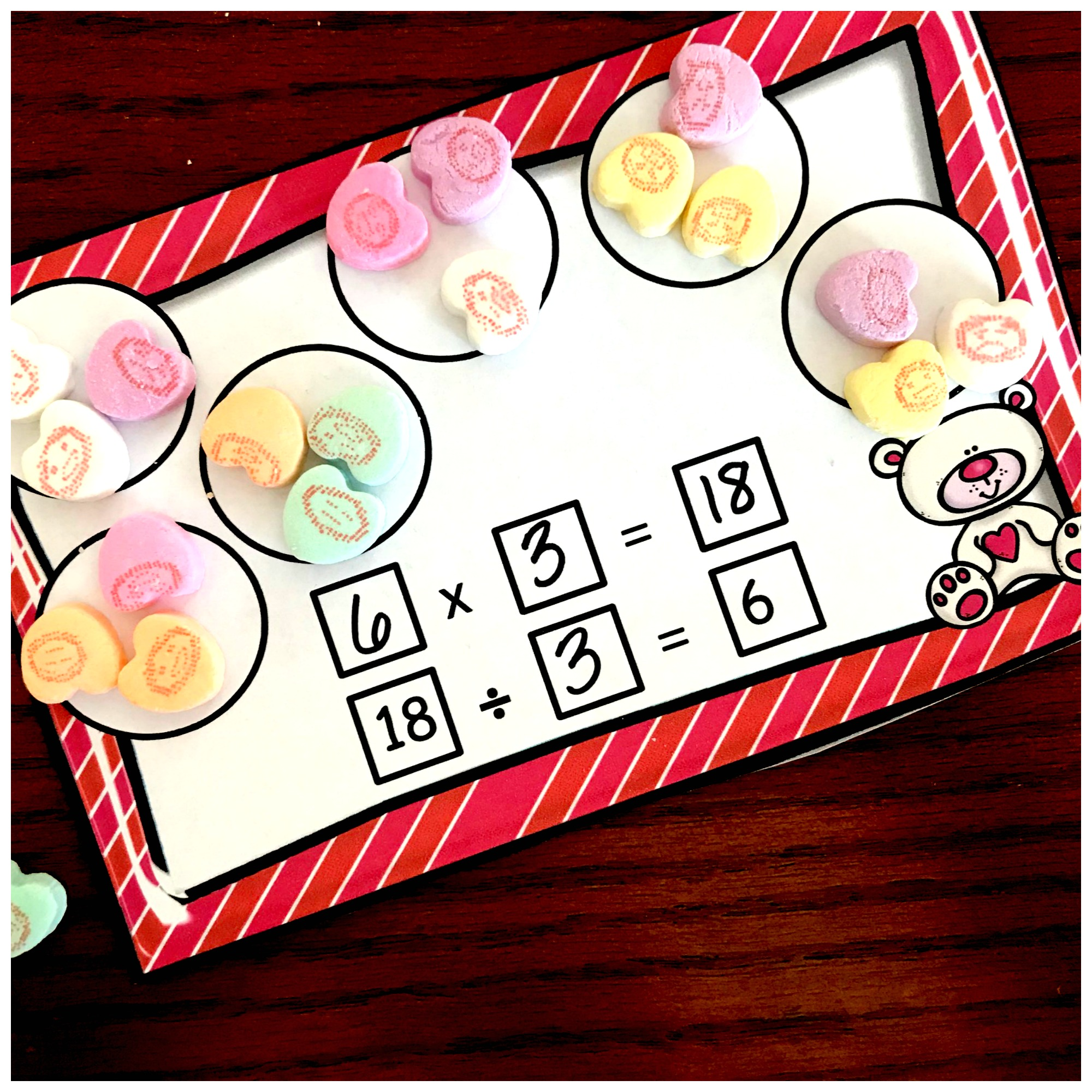Home » Math » Here’s FREE Missing Factor Worksheets or Task Cards For Extra Practice

Here’s FREE Missing Factor Worksheets or Task Cards For Extra Practice

These missing factor worksheets or task cards use conversation hearts to help children solve equations with missing factors, dividends, and divisors.Math was not easy for me in high school. I didn’t understand place value, and absolutely no fraction sense, and sat through most of the geometry classes in a horrible state of confusion. For some reason, I didn’t hate algebra class though. Maybe it was the teacher, or that I understood the steps better, but I had some success in this class.

High school algebra seems far off for us teaching young elementary children, but years fly by quickly. And what we do in our classrooms now, set the basis for how our students when they hit high school.

These missing factor worksheets or task cards are one way you can begin to build a strong foundation for your kiddos.

Get missing numbers for addition and subtraction herePrep Work –

The prep-work for these task cards are about the same as most task cards.

• First, print off task cards on card stock
• Next, cut out and laminate
• Finally, gather up conversation hearts and dry erase markers

Or make it like a worksheet by placing them in sheet protectors!

Missing Factor Worksheets Directions:

For the simple directions, Children just use the conversation hearts and circles to model the expressions and find the missing number.

But let’s look at some examples:In this example, the children were given the equation 3 x ___ = 6. When the children look at this there are two things they must understand.

First, they must realize that the product (or answer) is the total number of hearts that will be used.  Next, they must look at the one factor given and understand that this is how many groups will be used.

In this example, the product is 6……so the children need to get out six hearts.

Next, we see that one of the factors is 3. All this means is that we are going to take the six hearts, and put them into three groups. It should be pointed out that the groups MUST be equal.

As you can see, when is put into three equal groups, there will be 2 hearts in each group. Therefore….

3 x 2 = 6

6 ÷ 3 = 2Missing Dividends or Divisors

Other task cards start with division problems. Once again children must understand each part of a division equation, and this interactive book is a perfect way to learn this concept.

Once they understand all about divisor and dividends, it is time to get to work.

First, children need to look at the dividend and count out that many hearts….so 18 in this problem.

Next, they must divide those hearts up based on the quotation….in this problem they will divide them up into six equal groups.

Once this is done, they will see that ……

18 ÷ 3 = 6

6 x 3 = 6

As children work through these missing factor worksheets they will begin to develop a great understanding on division and multiplication, as well as build a strong foundation for algebra.

You’ve Got This

Rachel

You can get all my conversation heart activities here!